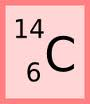Quiz: Atomic Structure & Atomic Mass Calculations

17 Questions | Total Attempts: 3080SettingsChoose or type the answer that best completes each question or prompt.

• 1.
The diagram below represents carbon-14. What is the mass number of carbon-14?
• A.

6

• B.

14

• C.

8

• D.

20

• 2.
The diagram below represents carbon-14. What is the atomic number of carbon-14?
• A.

6

• B.

14

• C.

8

• D.

20

• 3.
The diagram below represents carbon-14. How many neutrons are in an atom of carbon-14?
• A.

6

• B.

14

• C.

8

• D.

20

• 4.
An atom that has 8 protons, 8 neutrons, and 7 electrons would be considered an oxygen ion.
• A.

True

• B.

False

• 5.
An atom with 9 protons, 10 neutrons, and 9 electrons would be considered an isotope of fluorine.
• A.

True

• B.

False

• 6.
An atom that contains an unequal number of protons and electrons is called a(n)
• A.

Atom

• B.

Molecule

• C.

Isotope

• D.

Ion

• 7.
An atom that contains a different number of neutrons than the normally occurring version is called a(n)
• A.

Atom

• B.

Molecule

• C.

Ion

• D.

Isotope

• 8.
Which type of subatomic particle has a positive charge?
• A.

Proton

• B.

Neutron

• C.

Electron

• 9.
Which type of subatomic particle has a negative charge?
• A.

Proton

• B.

Neutron

• C.

Electron

• 10.
Which type of subatomic particle has no charge, meaning it is neutral?
• A.

Proton

• B.

Neutron

• C.

Electron

• 11.
In the diagram below, what color represents the electrons?
• A.

Blue

• B.

Yellow

• C.

Red

• D.

They are not represented.

• 12.
Which subatomic particle determines the identity of an atom? In other words, all atoms of one element will always have the same number of these.
• A.

Proton

• B.

Neutron

• C.

Electron

• 13.
Ga-69 has a mass of 68.926 amu and an abundance of 60.10%. Ga-71 has a mass of 70.925 amu and an abundance of 39.90%. What is the weighted average atomic mass of galium? (Type only a numeric answer. Do not include units.)
• 14.
Cl-35 has a mass of 34.969 amu and a relative abundance of 75.7%. Cl-37 has a mass of 36.965 amu and a relative abundance of 24.3%. What is the weighted average atomic mass of chlorine? (Type only a numeric answer. Do not include units.)
• 15.
Ag-107 has a mass of 106.905 amu and an abundance of 51.84%. Ag-109 has a mass of 108.905 and an abundance of 48.16%. What is the weighted average atomic mass of silver? (Type only a numeric answer. Do not include units.)
• 16.
Which particle(s) are found in the nucleus?
• A.

Proton

• B.

Neutron

• C.

Electron

• 17.
Which particle(s) are located outside the nucleus?
• A.

Proton

• B.

Neutron

• C.

Electron

Related TopicsBack to top